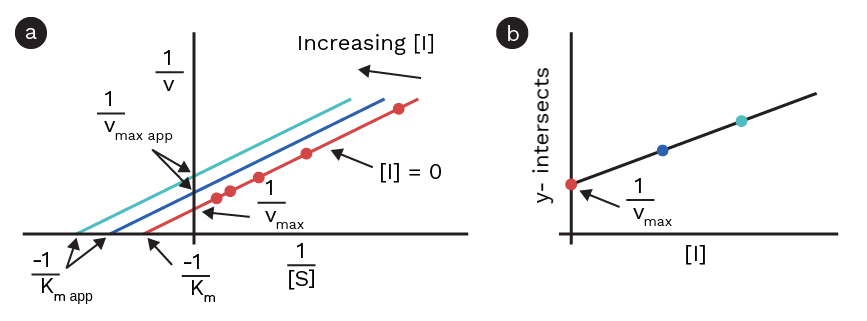An uncompetitive inhibitor interacts with the enzyme-substrate complex, but not with the enzyme alone. For uncompetitive inhibition, the double-reciprocal equation is as follows:

1/V0 = α’/Vmax + Km/Vmax • 1/[S]

where α’ = 1+[I]/K’I

This equation shows that a double-reciprocal plot of enzyme kinetic data with varying concentrations of an uncompetitive inhibitor should give a straight line with varying y-intercepts, but with the same slopes (Figure 1). In enzyme kinetic assays with an uncompetitive inhibitor, the apparent Km and Vmax will change with increasing inhibitor concentrations .Figure 1: a) Lineweaver-Burk plot showing the uncompetitive inhibition. b) y-intercepts of each linear regression plotted against the inhibitor concentration.

## Calculating K’I, Km and Vmax

When working with an uncompetitive inhibitor, no parameters can be calculated from the initial Lineweaver-Burk plots. To calculate the different parameters, the ‘’y-intercepts’’ of these plots must be plotted against the inhibitor concentration:

y-intersectuncompettive = α’/Vmax = 1/Vmax + 1/Vmax • 1/KI • [I]

This plot should, therefore, result in a straight line with the intercept 1/max and slope 1 / (Vmax • KI ). KI can, therefore, be calculated by dividing the slope of this straight line with the intercept. Vmax can also be calculated from this fit, by taking the reciprocal to the y-intercept. Because the initial Lineweaver-Burk plots had the same slopes, Km / Vmax, Km can now be calculated by multiplying these slopes with Vmax obtained from the plot of y-intercepts against inhibitor concentrations.

## Steps of calculating the kinetic parameters when using an uncompetitive inhibitor

• Prepare Lineweaver-Burk plots of the kinetic data and fit the data using linear regression (1 fit per inhibitor concentration).

• Plot the y-intercepts of each of these fits as a function of the inhibitor concentration.

• To calculate Vmax, take the reciprocal of the y-intercept of this plot.

• To calculate KI, divide the y-intercept of this plot with the slope.

• To calculate Km, go back to the first Lineweaver-Burk plots, take the slope from 1 of these plots (the slope should be the same, or close, for all the plots), and multiply it with Vmax.

1. Lehninger, Albert L.; Nelson, David L.; Cox, Michael M. (2008). Principles of Biochemistry (5th ed.). New York, NY: W.H. Freeman and Company. ISBN 978-0-7167-7108-1.

Theory overview